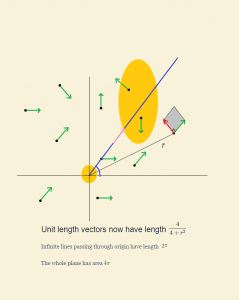# What is a Manifold? (4/6)

After our luxurious treatment of 1-d manifolds, we turn to 2-d manifolds.

My story of surfaces starts in a beautifully weird morning when I got up to realize that life in the usual Euclidean plane had changed dramatically. Vectors had shortened, areas had shrunk, and infinity was just a few feet away!

Here is the aftermath (why the term “after math?!”):What had happened was this: A vector of unit length in the usual sense was now much shorter, of length$\frac{4}{4+r^2}$ where$r$ is the distance (in usual sense) of its base (=initial point) from the origin. Yes, here it does matter where the base of a vector is.

The vectors that used to be perpendicular are still perpendicular. By looking at parallelograms, we see that areas have shrunk by a factor of$\frac{4}{4+r^2}$. So, in the above diagram, the square feet of the two shaded areas are in fact almost equal.

Let me do some measurements.

Length of an infinite straight ray.

Consider$\gamma (t)= (\cos \theta t, \sin \theta t); \ 0\leq t <\infty$ (see picture.) To calculate lengths we must add up lengths of tangent vectors. Tangent vectors are all$(\cos \theta , \sin \theta )$, unit length in ordinary plane at each point, so their new length must be taken into account. The result is:$\ l (\gamma) = \int _0^\infty \|\gamma ' (t) \|_{new} dt = \int _0^\infty \frac{4}{4+t^2}dt = 2tg^{-1}(t/2) |_{0}^\infty = \pi .$

Hmm! Seems that the infinity is only$\pi$ feet away!

The area of the whole plane! (But wait, isn’t that infinite?)

We add up areas of infinitesimal rectangles$dxdy$. As discussed above, in our new metric (Ok, I had to finally use the term!) this rectangle’s area is shrunk by our factor. So, we get$\int \int_{\mathcal{R}^2} \frac{4}{4+r^2} dxdy = 4 \pi.$

Maybe my whole success in posting these series depends on my ability to convince you now to experiment with this new plane I have created. Be bold about it. Ask questions, do calculations for yourself, get a feel of the structure of this space. Ask what could be asked about this space? What other familiar constructions are possible on it? Could we talk about$L^p$ spaces of functions? What is the measure? How will we integrate a function? What is the shortest path, say between$(1,0)$ and$(0,1)$? Notice that going on the straight line isn’t the best, because we are better off bending away from the origin so that lengths are shorter. But how much to bend?!

And finally answer this: the plane with this tampered metric is a copy (in almost all aspects) of a familiar 2-d shape, can you guess what shape?!

To be continued with more fun…## About Behnam Esmayli

I started PhD in Mathematics at Pitt in Fall 2015. I have come to grow a passion for metric spaces -- a set and a distance function that satisfies the triangle inequality -- simple and beautiful! These spaces when equipped with other structures, such as a measure, becomes extremely fun to play with!
This entry was posted in Math and tagged , . Bookmark the permalink.

### 3 Responses to What is a Manifold? (4/6)

1.Brandon says:

Hi, I’m confused. If the length of a unit vector depends on where the base is, doesn’t that mean that we could take one vector and call either end of it the “base,” which would give two different measurements for its length (provided the vector is not a secant of a circle centered at the origin)? Isn’t that, like, bad?

•Behnam Esmayli says:

Thanks for asking. The point here is that we do not identify any two vectors whose bases are different. It is the particular geometry of R^n that allows us to unambiguously translate a vector (parallel) to any initial point. In our new geometry, the vector i, with initial point at origin has nothing to do with i, centered at (1,1). If we switch initial and end points, the arising two vectors have NOTHING to do with each other. They simply belong to two different vector spaces.

•Brandon says:

Oh neat! Thank you!